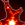{ "cells": [ { "cell_type": "code", "execution_count": 1, "metadata": {}, "outputs": [ { "name": "stdout", "output_type": "stream", "text": [ "Populated the namespace with:\n", "np, matplotlib, kwant, holoviews, init_notebook, SimpleNamespace, pprint_matrix, scientific_number, pretty_fmt_complex, plt, pf, display_html\n", "from code/edx_components:\n", "MoocVideo, MoocDiscussion, MoocCheckboxesAssessment, MoocMultipleChoiceAssessment, MoocSelfAssessment\n", "from code/functions:\n", "spectrum, hamiltonian_array, h_k, pauli\n", "Using kwant 1.3.2 and holoviews 1.7.0-x-gf3a9f4f\n", "Executed on 2019-01-31 at 20:43:44.966871.\n" ] }, { "data": { "text/html": [ "\n", "\n", "\n", "\n", "\n", "\n", "\n", "\n", "\n", "
\n", "\n", "\n", "\n", "
\n" ], "text/plain": [ "" ] }, "metadata": {}, "output_type": "display_data" } ], "source": [ "import sys\n", "sys.path.append('../code')\n", "from init_mooc_nb import *\n", "init_notebook()\n", "\n", "%opts Layout [sublabel_format='' aspect_weight=1 figure_size=(100) vspace=0.4]\n", "%output size = 150\n", "\n", "randn = np.random.randn\n", "\n", "alphas = np.linspace(0, 1, 1000)\n", "\n", "dims = SimpleNamespace(E=holoviews.Dimension(r'$E$'),\n", " alpha=holoviews.Dimension(r'$\\alpha$'),\n", " Q=holoviews.Dimension(r'$Q$'),\n", " Q_BdG=holoviews.Dimension(r'$Q_{BdG}$'))\n", "\n", "def make_random_real_ham(N):\n", " H = randn(N, N)\n", " H += H.T\n", " return H / 2\n", "\n", "\n", "def make_cons_ham(N):\n", " H = np.kron(pauli.s0, randn(N, N)) + np.kron(pauli.sz, randn(N, N))\n", " H += H.T.conj()\n", " return H / 2\n", "\n", "\n", "def make_random_ham(N):\n", " H = randn(N, N) + 1j * randn(N, N)\n", " H += H.T.conj()\n", " return H / 2\n", "\n", "\n", "def make_random_symplectic_ham(N):\n", " if N % 2:\n", " raise ValueError('Matrix dimension should be a multiple of 2')\n", " sy = np.kron(np.eye(N // 2), np.array([[0, -1j], [1j, 0]]))\n", " h = randn(N, N) + 1j * randn(N, N)\n", " h += h.T.conj()\n", " Th = sy @ h.conj() @ sy\n", " return (h + Th) / 4\n", "\n", "\n", "def make_chiral_ham(N):\n", " temp1 = randn(N, N) + 1j * randn(N, N)\n", " temp2 = randn(N, N) + 1j * randn(N, N) \n", " H = np.kron(pauli.sx, temp1) + np.kron(pauli.sy, temp2)\n", " H += H.T.conj()\n", " return H / 2\n", "\n", "\n", "def make_BdG_ham(N):\n", " # This is antisymmetric basis\n", " H = 1j * randn(2*N, 2*N)\n", " H += H.T.conj()\n", " return H / 2\n", "\n", "\n", "def energies(alpha, H0, H1):\n", " H = (1 - alpha) * H0 + alpha * H1\n", " return np.linalg.eigvalsh(H)\n", "\n", "\n", "def find_spectrum(alphas, H0, H1):\n", " spectrum = [energies(a, H0, H1) for a in alphas]\n", " return np.array(spectrum)\n", "\n", "\n", "def find_Q(spectrum):\n", " \"\"\"Finds the number of bands that are under zero energy.\n", "\n", " Parameters:\n", " -----------\n", " spectrum : numpy array\n", " Array that contains the energies levels for every alpha.\n", "\n", " Returns:\n", " --------\n", " Q : list\n", " Number of bands under zero energy.\n", " \"\"\"\n", " return [len(s[s<0]) for s in spectrum]\n", "\n", "\n", "def plot_hamiltonian_spectrum(alphas, spectrum, E_range=(-4, 4)):\n", " \"\"\"Function that plots a spectrum for a range of alphas.\n", "\n", " Parameters:\n", " -----------\n", " alphas : numpy array\n", " Range of alphas for which the energies are calculated.\n", " spectrum : numpy array\n", " Array that contains the energies levels for every alpha.\n", " E_range : tuple\n", " The upper and lower limit of the y-dimension.\n", "\n", " Returns:\n", " --------\n", " plot : holoviews.Path object\n", " Plot of alphas vs. spectrum.\n", " \"\"\"\n", " E_min, E_max = E_range\n", " plot = holoviews.Path((alphas, spectrum), kdims=[dims.alpha, dims.E])[:, E_min:E_max] * holoviews.HLine(0)\n", " return plot(plot={'xticks':[0, 0.5, 1], 'yticks':[E_min, 0, E_max]})\n", "\n", "\n", "def plot_Q(alphas, Q, Q_range, Q_dim=dims.Q):\n", " \"\"\"Function that plots value of Q for a range of alphas.\n", "\n", " Parameters:\n", " -----------\n", " alphas : numpy array\n", " Range of alphas for which the energies are calculated.\n", " Q : numpy array\n", " Vector that contains the value of Q for every alpha.\n", " Q_range : tuple\n", " The upper and lower limit of the y-dimension.\n", "\n", " Returns:\n", " --------\n", " plot : holoviews.Path object\n", " Plot of alphas vs. Q.\n", " \"\"\"\n", " Q_min, Q_max = Q_range\n", " Q_mid = (Q_max + Q_min) / 2\n", " plot = holoviews.Area((alphas, Q), kdims=[dims.alpha], vdims=[Q_dim])[:, Q_min:Q_max](style={'alpha': 0.4})\n", " plot *= holoviews.Curve((alphas, Q), kdims=[dims.alpha], vdims=[Q_dim])[:, Q_min:Q_max]\n", " return plot(plot={'xticks':[0, 0.5, 1], 'yticks':[Q_min, Q_mid, Q_max], 'aspect':4})" ] }, { "cell_type": "markdown", "metadata": {}, "source": [ "# Topology and symmetry" ] }, { "cell_type": "code", "execution_count": 2, "metadata": {}, "outputs": [ { "data": { "application/vnd.edx.olxml+xml": "